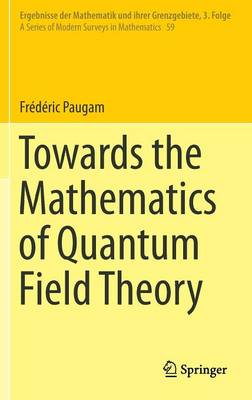•Towards the Mathematics of Quantum Field Theory - Ergebnisse der Mathematik und ihrer Grenzgebiete. 3. Folge / A Series of Modern Surveys in Mathematics 59 (Hardback)

(author)
£129.99
Hardback 487 Pages / Published: 05/03/2014
• We can order this from the publisher

Usually dispatched within 15 working days

This ambitious and original book sets out to introduce to mathematicians (even including graduate students ) the mathematical methods of theoretical and experimental quantum field theory, with an emphasis on coordinate-free presentations of the mathematical objects in use. This in turn promotes the interaction between mathematicians and physicists by supplying a common and flexible language for the good of both communities, though mathematicians are the primary target. This reference work provides a coherent and complete mathematical toolbox for classical and quantum field theory, based on categorical and homotopical methods, representing an original contribution to the literature.
The first part of the book introduces the mathematical methods needed to work with the physicists' spaces of fields, including parameterized and functional differential geometry, functorial analysis, and the homotopical geometric theory of non-linear partial differential equations, with applications to general gauge theories. The second part presents a large family of examples of classical field theories, both from experimental and theoretical physics, while the third part provides an introduction to quantum field theory, presents various renormalization methods, and discusses the quantization of factorization algebras.

Publisher: Springer International Publishing AG
ISBN: 9783319045634
Number of pages: 487
Weight: 8749 g
Dimensions: 235 x 155 x 27 mm
Edition: 2014 ed.

MEDIA REVIEWS

"The book under review is a valuable witness to a wide range of recent developments that aim at unraveling the mathematics of quantum field theory. ... the book is well written with an original organization of the material. Its reductionist viewpoint makes it a valuable textbook, especially for the more experienced practitioners in this domain of mathematical physics." (Walter D. van Suijlekom, Jahresbericht der Deutschen Mathematiker-Vereinigung, Vol. 117, 2015)

"This is a highly specialized monograph exposing current research in a systematic way, aimed at researchers and graduate students in this area. The author has tried to include several examples to illustrate the general methods being treated and ease the reader through the journey." (Felipe Zaldivar, MAA Reviews, July, 2014)

"This is a complete guide to the mathematics underlying the laws of physics in general and quantum physics in particular. ... Frederic Paugam has given us a work of enormous scope and shows students a road to a coordinate-free presentation of mathematical objects underlying classical and quantum field theories. ... The book will be useful to graduate student, but also to pure and applied mathematicians interested in modern mathematical developments related to quantum theory." (Gert Roepstorff, zbMATH, Vol. 1287, 2014)